Next: SLA_AV2M - Rotation Matrix from Axial Vector
Up: SUBPROGRAM SPECIFICATIONS
Previous: SLA_AOPQK - Quick Appt-to-Observed

## SLA_ATMDSP - Atmospheric Dispersion

ACTION:
Apply atmospheric-dispersion adjustments to refraction coefficients.
CALL:
CALL sla_ATMDSP (TDK, PMB, RH, WL1, A1, B1, WL2, A2, B2)

GIVEN:

 D TDK ambient temperature at the observer (degrees K) PMB D pressure at the observer (mB) RH D relative humidity at the observer (range 0-1) WL1 D base wavelength (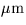) A1 D refraction coefficient A for wavelength WL1 (radians) B1 D refraction coefficient B for wavelength WL1 (radians) WL2 D wavelength for which adjusted A,B required ()

RETURNED:

 D A2 refraction coefficient A for wavelength WL2 (radians) B2 D refraction coefficient B for wavelength WL2 (radians)

NOTES:
1.
To use this routine, first call sla_REFCO specifying WL1 as the wavelength. This yields refraction coefficients A1, B1, correct for that wavelength. Subsequently, calls to sla_ATMDSP specifying different wavelengths will produce new, slightly adjusted refraction coefficients A2, B2, which apply to the specified wavelength.
2.
Most of the atmospheric dispersion happens between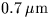and the UV atmospheric cutoff, and the effect increases strongly towards the UV end. For this reason a blue reference wavelength is recommended, for example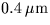.
3.
The accuracy, for this set of conditions:

 height above sea level 2000m latitude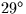pressure 793mB temperature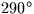K humidity 0.5 (50%) lapse rate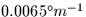reference wavelengthstar elevation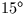is about 2.5mas RMS between 0.3 and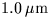, and stays within 4mas for the whole range longward of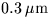(compared with a total dispersion from 0.3 to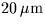of about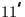). These errors are typical for ordinary conditions; in extreme conditions values a few times this size may occur.
4.
If either wavelength exceeds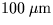, the radio case is assumed and the returned refraction coefficients are the same as the given ones.
5.
The algorithm consists of calculation of the refractivity of the air at the observer for the two wavelengths, using the methods of the sla_REFRO routine, and then scaling of the two refraction coefficients according to classical refraction theory. This amounts to scaling the A coefficient in proportion to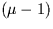and the B coefficient almost in the same ratio (see R.M.Green, Spherical Astronomy, Cambridge University Press, 1985).Next: SLA_AV2M - Rotation Matrix from Axial Vector
Up: SUBPROGRAM SPECIFICATIONS
Previous: SLA_AOPQK - Quick Appt-to-Observed

SLALIB --- Positional Astronomy Library